# 起动射流出口形状对其推力特性影响研究 Effects of Exit Geometry on the Propulsive Characteristics of the Starting Jet

DOI: 10.12677/APP.2019.94024, PDF, HTML, XML, 下载: 508  浏览: 799

Abstract: The effect of the exit geometry of a vortex ring generator on the propulsive characteristics of the starting jet was studied numerically. For the orifice and tube nozzle configurations, the ratio of the nozzle entrance to exit diameters, dubbed as the diameter ratio Dr, was found to have significant effect on propulsive characteristics of the starting jet. The higher impulse generated by the orifice nozzle (Dr > 1) than that of the tube nozzle (Dr = 1) is attributed to the consistent over-pressure effect at the nozzle exit plane during the entire fluid ejection history, while the over-pressure effect only exist during the initial jet startup for the tube nozzle. The results show that the hydrodynamic impulse and net propulsive impulse of the starting jet increases against the diameter ratio until a critical value of around Dr = 2.5 is approached.

1. 引言

${t}^{*}=\stackrel{¯}{{U}_{p}}t/D$，其中 $\stackrel{¯}{{U}_{p}}$ 为活塞推进平均速度。他们发现，在形成时间达到某个值后，前导涡环开始与尾迹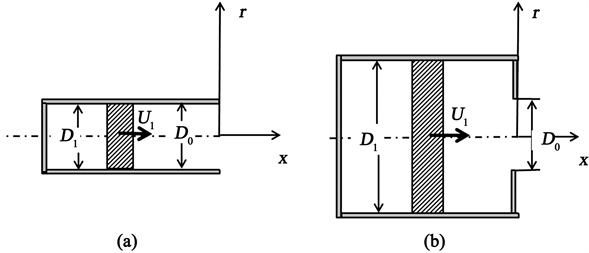Figure 1. Schematic of (a) tube nozzle and (b) orifice type piston-cylinder vortex ring generations

2. 数值模拟方法及验证

2.1. 计算方法及边界条件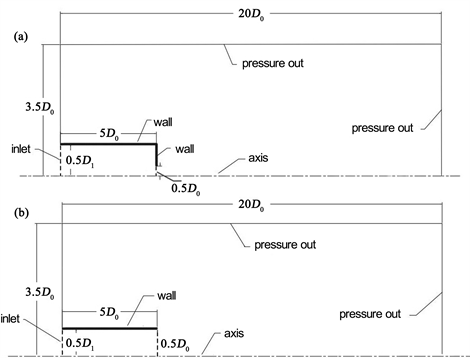Figure 2. Domains and condition used in numerical simulation of vortex ring formation: (a) Tube nozzle; (b) Orifice nozzle. Note that the sketches are not drawn in proportion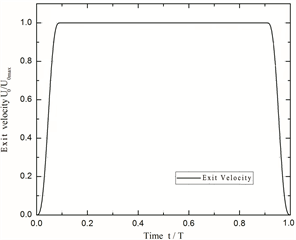Figure 3. Exit velocity programs used to generate the starting jets

2.2. 数值结果验证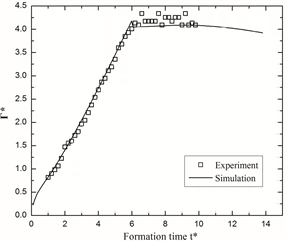Figure 4. Comparison of the total circulation of the numerical and experimental results

3. 结果及讨论

3.1. 起动射流动力学特征参数的变化

$I=\text{π}\rho {\int }^{\text{​}}\omega {r}^{2}\text{d}x\text{d}r$ (1)

$\Gamma ={\int }^{\text{​}}\omega \text{d}x\text{d}r$ (2)

$\frac{\text{d}\Gamma }{\text{d}t}=\frac{1}{2}{u}_{0}^{2}$ (3)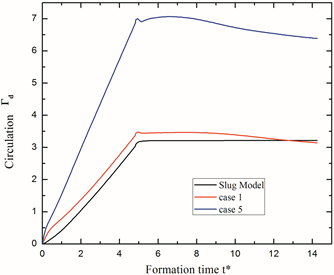Figure 5. Evolution of dimensionless total circulation with Dr = 1 (case 1) and Dr = 2.5 (case 5)

$\frac{\text{d}\Gamma }{\text{d}t}=\frac{1}{2}{u}_{0}^{2}+{\int }_{0}^{{R}_{\infty }}u\frac{\partial v}{\partial x}\text{d}r$ (4)

Dr = 1和2.5的另一个动力特征参数，水力冲量的分析如图6所示。图中对水力冲量进行了无量纲化，即 ${I}_{d}={I}_{h}/\text{π}\rho {D}_{0}^{3}{U}_{0\mathrm{max}}$ 。与环量在加速阶段的发展相似，射流的水力冲量由于射流加速度的作用，在运动初期会有小幅度的陡升，然后线性上升直至射流减速阶段。当达到t* = 5左右时，起动射流开始减速至停止，射流减速作用使冲量陡降较小幅度。然后，随着无量纲形成时间的增加，水力冲量以很小的幅度上升。在大冲程比的情况下(本文冲程比 $L/D\approx 5$ )，圆管喷管的出口过压所产生的冲量只在射流加速阶段较为明显，而在匀速阶段，超压效应几乎可以忽略   ，因此对于圆管来说，Slug模型虽然忽略了出口过压所产生的冲量值，但是仍然能够很好的描述水力冲量。但是对于圆孔装置而言，射流出口收缩效应导致出口过压产生的额外冲量一直存在，因此Slug模型忽略压力效应造成的冲量误差也就更大，不能很好的描述圆孔装置环量和水力冲量的发展。这一结果也与Krieg和Mohseni  的研究相符。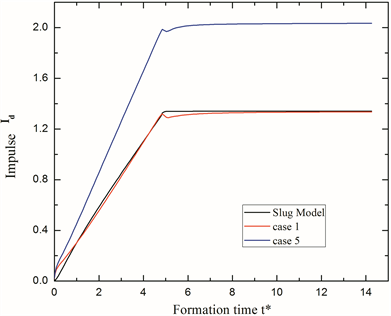Figure 6. Evolution of dimensionless hydrodynamic impulse with Dr = 1 (case 1) and Dr = 2.5 (case 5)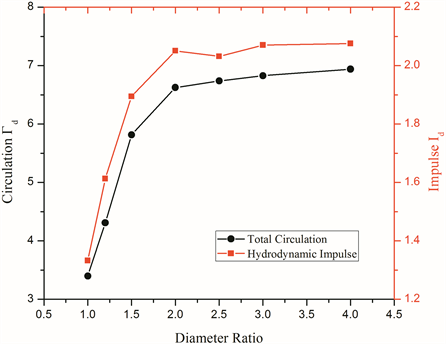Figure 7. Evolution of dimensionless total circulation and hydrodynamic impulse with different diameter ratios

3.2. 出口超压效应对射流的影响

${I}_{h}\left(t\right)={I}_{U}\left(t\right)+{I}_{p}\left(t\right)$ (5)

${I}_{U}\left(t\right)=\rho {\int }_{0}^{t}{\int }_{A}{u}_{0}^{2}\left(r,\tau \right)\text{d}S\text{d}\tau$ (6)

${I}_{p}\left(t\right)={\int }_{0}^{t}{\int }_{A}\left[{p}_{0}\left(r,\tau \right)-{p}_{\infty }\right]\text{d}S\text{d}\tau$ (6)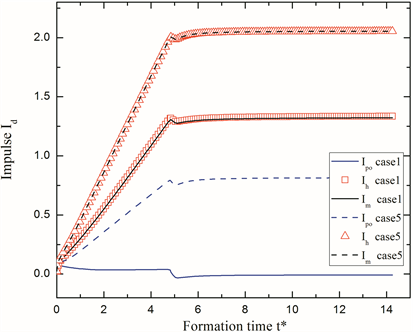Figure 8. The comparison between pressure impulse and momentum flux of exit and total hydrodynamic impulse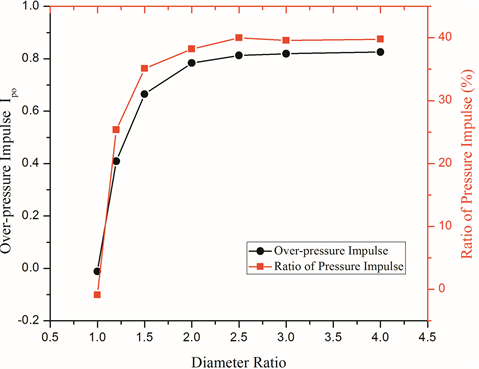Figure 9. The pressure impulse and the ratio of the pressure impulse to the hydrodynamic impulse for starting jets with different diameter ratios

3.3. 起动射流的推力冲量变化

${F}_{T}={F}_{pi}+{F}_{mi}+{F}_{pwo}-{F}_{pwi}$ (8)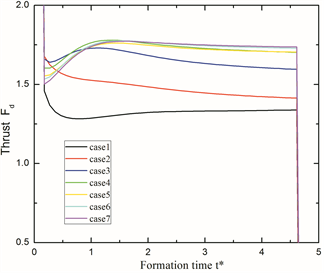(a)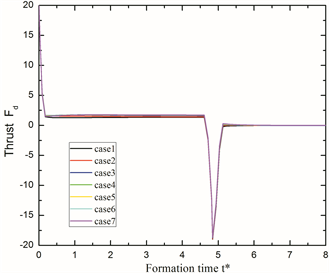(b)

Figure 10. Evolution of net propulsive thrust of starting jet in (a) the whole jet phase (b) the uniform velocity phase for different diameter ratio

4. 总结

NOTES

*通讯作者。

  Gharib, M., Rambod, E. and Shariff, K. (1998) A Universal Time Scale for Vortex Ring Formation. Journal of Fluid Mechanics, 360, 121-140.  Maxworthy, T. (1977) Some Experimental Studies of Vortex Rings. Journal of Fluid Mechanics, 81, 465-495.  Krueger, P.S. and Gharib, M. (2003) The Significance of Vortex Ring Formation to the Impulse and Thrust of a Starting Jet. Physics of Fluids, 15, 1271.  Pullin, D.I. (1979) Vortex Ring Formation at Tube and Orifice Openings. Physics of Fluids, 22, 401-403.  Olcay, A.B. and Krueger, P.S. (2008) Measurement of Ambient Fluid Entrainment during Laminar Vortex Ring Formation. Experiments in Fluids, 44, 235-247.  Krueger, P.S. and Gharib, M. (2005) Thrust Augmentation and Vortex Ring Evolution in a Fully-Pulsed Jet. AIAA Journal, 43, 792-801.  Krueger, P.S. (2006) Circulation of Vortex Rings Formed From Tube and Orifice Openings. Asme Joint US-European Fluids Engineering Summer Meeting Collocated with the International Conference on Nuclear Engineering, American Society of Mechanical Engineers.  Krueger, P.S. (2008) Circulation and Trajectories of Vortex Rings Formed from Tube and Orifice Openings. Physica D, 237, 2218-2222.  James, S. and Madnia, C.K. (1996) Direct Numerical Simulation of a Laminar Vortex Ring. Physics of Fluids, 8, 2400-2414.  Michael, K. and Kamran, M. (2013) Modelling Circulation, Impulse and Kinetic Energy of Starting Jets with Non-Zero Radial Velocity. Journal of Fluid Mechanics, 719, 488-526.  Krueger, P.S. and Olcay, A.B. (2010) Momentum Evolution of Ejected and Entrained Fluid during Laminar Vortex Ring Formation. Theoretical & Computational Fluid Dynamics, 24, 465-482.